﻿ 基于Sinc函数的回归算法

# 基于Sinc函数的回归算法Sinc Function-Based Regression Algorithm

Abstract: In this paper, the reconstruction of discrete signals in sampling theorem is introduced, and a linear and logistic regression model based on Sinc function is proposed. In order to minimize the mean square error of the regression function, Sinc function is designed in the independent variable do-main of data, and then the regression curve is reconstructed directly. On the basis of the linear and logistic regression model based on Sinc function and the algorithm analysis, a sufficient simulation experiment is carried out. And compared with the traditional linear regression, regression algorithm based on Sinc function has obvious advantages when the regression function is not obvious. Finally, the linear regression algorithm based on Sinc function is succeeding to predict the monthly minimum temperature.

1. 引言

2. 回归模型

2.1. 基于Sinc的线性回归

2.1.1. 代价函数最小化

${x}^{\left(k\right)}$ 上的代价函数为：

$J{\left(\theta \right)}^{k}=\frac{1}{p}\underset{i=1}{\overset{p}{\sum }}\frac{1}{2}{\left({h}_{\theta }\left({x}^{\left(k\right)}\right)-{y}_{k}^{\left(i\right)}\right)}^{2}$ (1)

1) 中 ${h}_{\theta }\left({x}^{\left(k\right)}\right)$ 表示最终的回归函数在 ${x}^{\left(k\right)}$ 上对应因变量的值。

$\begin{array}{l}\frac{\text{d}J{\left(\theta \right)}^{k}}{\text{d}{h}_{\theta }\left({x}^{\left(k\right)}\right)}=\frac{1}{p}\underset{i=1}{\overset{p}{\sum }}\left({h}_{\theta }\left({x}^{\left(k\right)}\right)-{y}_{k}^{\left(i\right)}\right)={h}_{\theta }\left({x}^{\left(k\right)}\right)-\frac{\underset{i=1}{\overset{p}{\sum }}{y}_{k}^{\left(i\right)}}{p}=0\\ {h}_{\theta }\left({x}^{\left(k\right)}\right)=\frac{\underset{i=1}{\overset{p}{\sum }}{y}_{k}^{\left(i\right)}}{p}={y}_{k}\end{array}$ (2)

$x\left[n\right]={y}_{k}\text{\hspace{0.17em}}\left(n=k=1,2,3,\cdots \right)$ (3)

2.1.2. 基于低通滤波的时域重构

F的傅里叶变换为：

$F\left(f\right)=\int {\text{e}}^{-2\text{π}j\left(x\Omega \right)}f\left(x\right)\text{d}x$ (4)

${T}_{s}$ 表示数据集中自变量t间的最小间隔， ${x}_{a}\left(t\right)$ 为最优曲线 $f\left(x\right)$ 的原函数。

${\delta }_{T}\left(t\right)=\underset{n=-\infty }{\overset{\infty }{\sum }}\delta \left(t-nT\right)$ (5)

${x}_{d}\left(t\right)={x}_{a}\left(t\right){\delta }_{T}\left(t\right)=\underset{n=-\infty }{\overset{\infty }{\sum }}{x}_{a}\left(nT\right)\delta \left(t-nT\right)$ (6)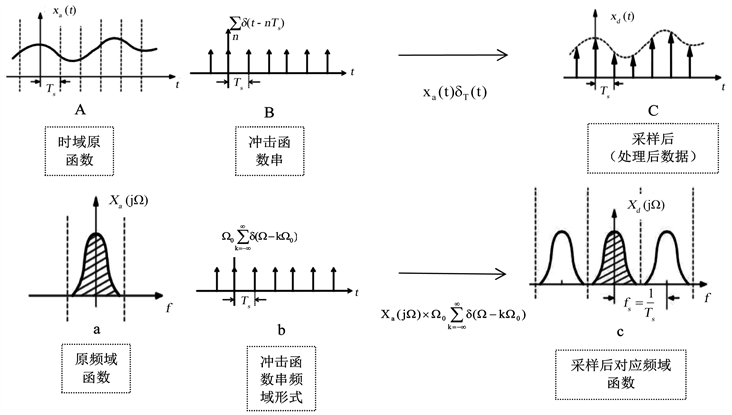Figure 1. Sampling process

${X}_{d}\left(j\Omega \right)=\frac{1}{{T}_{s}}\underset{k=-\infty }{\overset{\infty }{\sum }}{X}_{a}\left(j\left(\Omega -k{\Omega }_{0}\right)\right)$ (7)

$\frac{{\Omega }_{s}}{2}$ 可以包含最优函数频域的主频范围。低通滤波器的频域表达式如下：

${H}_{r}\left(j\Omega \right)=\left\{\begin{array}{l}T,\text{\hspace{0.17em}}\text{\hspace{0.17em}}\text{\hspace{0.17em}}|\Omega |\le {\Omega }_{s/2}\\ 0,\text{\hspace{0.17em}}\text{\hspace{0.17em}}\text{\hspace{0.17em}}\text{ }|\Omega |>{\Omega }_{s/2}\end{array}$

${\Omega }_{\frac{s}{2}}=\frac{\text{π}}{{T}_{s}}$

$\begin{array}{c}{x}_{a}\left(t\right)={x}_{d}\left(t\right)\ast {h}_{r}\left(t\right)=\underset{n=-\infty }{\overset{\infty }{\sum }}{x}_{a}\left(n{T}_{s}\right){h}_{r}\left(t-n{T}_{s}\right)\\ =\underset{n=-\infty }{\overset{\infty }{\sum }}x\left[n\right]{h}_{r}\left(t-n{T}_{s}\right)\end{array}$ (8)

${h}_{r}\left(t\right)=\frac{1}{\text{2π}}{\int }_{-\infty }^{\infty }{H}_{r}\left(j\Omega \right){\text{e}}^{j\Omega t}\text{d}\Omega =\frac{T}{\text{2π}}{\int }_{-{\Omega }_{s}/2}^{{\Omega }_{s}/2}{\text{e}}^{j\Omega t}\text{d}\Omega =\frac{\mathrm{sin}\left({\Omega }_{s}t/2\right)}{{\Omega }_{s}t/2},\text{\hspace{0.17em}}\text{\hspace{0.17em}}\text{\hspace{0.17em}}\text{\hspace{0.17em}}\text{\hspace{0.17em}}\text{\hspace{0.17em}}-\infty \le t\le \infty$ (9)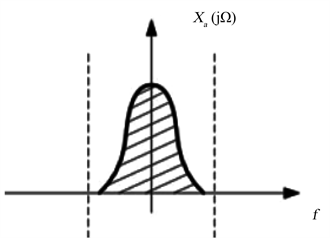Figure 2. Obtain the frequency domain function by the low pass filter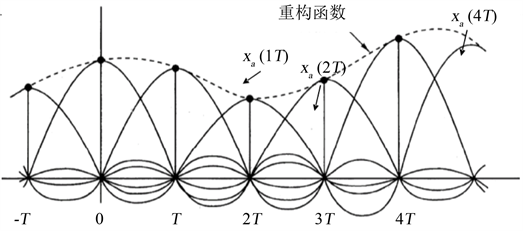Figure 3. Time domain reconstructionFigure 4. Sinc

2.1.3. 基于DTFT基本公式的时域重构

${x}_{a}\left(t\right)$ 的正反傅里叶变换：

$\begin{array}{l}{X}_{a}\left(j\Omega \right)=\underset{t=-\infty }{\overset{\infty }{\int }}{x}_{a}\left(t\right){\text{e}}^{\left(-j\Omega t\right)}\text{d}t\\ {x}_{a}\left(t\right)=\frac{1}{\text{2π}}\underset{\Omega =-\infty }{\overset{\infty }{\int }}X\left(\Omega \right){\text{e}}^{\left(j\Omega t\right)}\text{d}\Omega =\frac{1}{\text{2π}}\underset{\Omega =-{\Omega }_{0}}{\overset{{\Omega }_{0}}{\int }}X\left(\Omega \right){\text{e}}^{\left(j\Omega t\right)}\text{d}\Omega \end{array}$ (10)

x[n]的正反DTFT变换：

$\begin{array}{l}X\left(\omega \right)=\underset{n=-\infty }{\overset{\infty }{\sum }}x\left[n\right]{\text{e}}^{\left(-j\omega n\right)}\\ x\left[n\right]=\frac{1}{\text{2π}}\underset{\omega =-\text{π}}{\overset{\text{π}}{\int }}X\left(\omega \right){\text{e}}^{\left(j\omega n\right)}\text{d}\omega \end{array}$ (11)

$x\left[n\right]=\frac{1}{\text{2π}}\underset{\omega =-\text{π}}{\overset{\text{π}}{\int }}X\left(\omega \right){\text{e}}^{\left(j\omega n\right)}\text{d}\omega =\frac{1}{\text{2π}}\underset{\omega =-\text{π}}{\overset{\text{π}}{\int }}X\left(\Omega \right){\text{e}}^{\left(j\Omega {T}_{s}\right)}\text{d}\Omega {T}_{s}={T}_{s}x\left(n{T}_{s}\right)$ (12)

$X\left(\omega \right)=\frac{{X}_{a}\left(j\Omega \right)}{{T}_{s}}.$

$\begin{array}{c}{x}_{a}\left(t\right)=\frac{1}{\text{2π}}\underset{\Omega =-\infty }{\overset{\infty }{\int }}X\left(\Omega \right){\text{e}}^{\left(j\Omega t\right)}\text{d}\Omega \\ =\frac{{T}_{s}}{\text{2π}}\underset{{}_{\omega =-\text{π}}}{\overset{\text{π}}{\int }}X\left(\omega \right){\text{e}}^{\left(\frac{j\omega t}{{T}_{s}}\right)}\text{d}\frac{\omega }{{T}_{s}}\\ =\frac{{T}_{s}}{\text{2π}}\underset{{}_{\omega =-\text{π}}}{\overset{\text{π}}{\int }}\left(\underset{n=-\infty }{\overset{\infty }{\sum }}x\left[n\right]{\text{e}}^{\left(-j\omega n\right)}\right){\text{e}}^{\left(\frac{j\omega t}{{T}_{s}}\right)}\text{d}\frac{\omega }{{T}_{s}}\\ =\frac{{T}_{s}}{2\text{π}}\underset{n=-\infty }{\overset{\infty }{\sum }}x\left[n\right]\left(\underset{{}_{\omega =-\text{π}}}{\overset{\text{π}}{\int }}{\text{e}}^{\left(-j\omega n\right)}{\text{e}}^{\left(\frac{j\omega t}{{T}_{s}}\right)}\text{d}\frac{\omega }{{T}_{s}}\right)\\ =\underset{n=-\infty }{\overset{\infty }{\sum }}x\left[n\right]\frac{\mathrm{sin}\left(\left(t-n{T}_{s}\right)\frac{\text{π}}{{T}_{s}}\right)}{\left(t-n{T}_{s}\right)\frac{\text{π}}{{T}_{s}}}=\underset{n=-\infty }{\overset{\infty }{\sum }}x\left[n\right]{h}_{r}\left(t\right)\end{array}$ (13)

2.1.4. 基于Sinc函数的线性回归算法步骤

1) 求出每个自变量上的最优点，对y做如下操作：

for i = 1:N

${y}_{m×N}$ 第i列加和除以m，放入x[i]中

end for。

2) 将T带入公式(9)求出相应Sinc函数。

3) 最优点分别与4)中所得Sinc函数相乘并累加，得y。

4) 算法结束。

$T\left(n\right)=O\left(a+N\right)$ (14)

2.2. 基于Sinc的逻辑回归

2.2.1. 旋转

2.2.2. 代价函数最小化

$Cost\left({h}_{\theta }\left(x\right),y\right)=\left\{\begin{array}{l}-\mathrm{log}\left(1-{h}_{\theta }\left(x\right)\right),\text{\hspace{0.17em}}\text{\hspace{0.17em}}\text{if}\text{\hspace{0.17em}}y=0\\ -\mathrm{log}\left({h}_{\theta }\left(x\right)\right),\text{\hspace{0.17em}}\text{\hspace{0.17em}}\text{\hspace{0.17em}}\text{\hspace{0.17em}}\text{\hspace{0.17em}}\text{\hspace{0.17em}}\text{if}\text{\hspace{0.17em}}y=1\end{array}$ (15)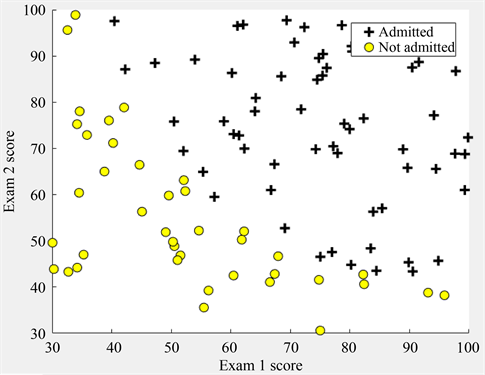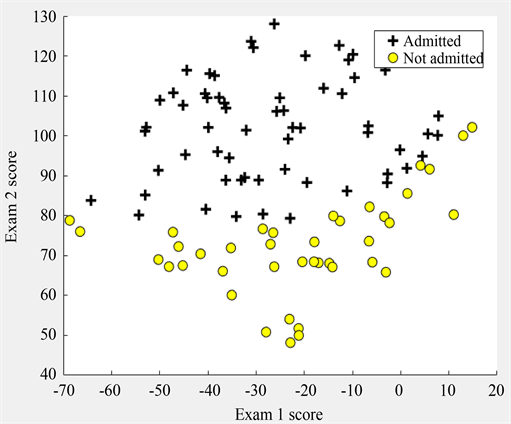Figure 6. Data rotated 60˚

$\begin{array}{l}{h}_{\theta }\left(x\right)=\text{sigmoid}\left(z\right)=\frac{1}{1+{\text{e}}^{-z}}\\ z={W}^{\text{T}}X\end{array}$ (16)

2.2.3. 确定边界函数

${\text{distince}}^{k}=\underset{i=1}{\overset{p}{\sum }}{\left({x}_{2}^{i}-{x}_{0}^{k}\right)}^{2}$ (17)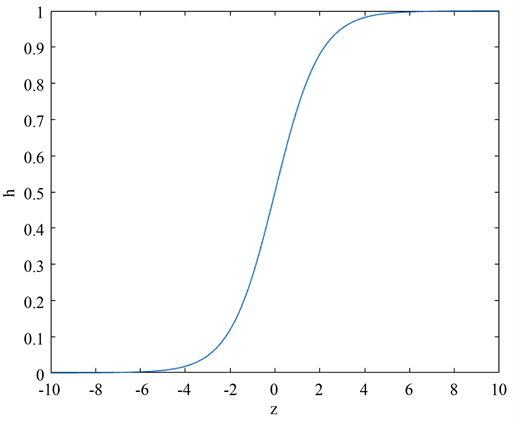Figure 7. Sigmoid function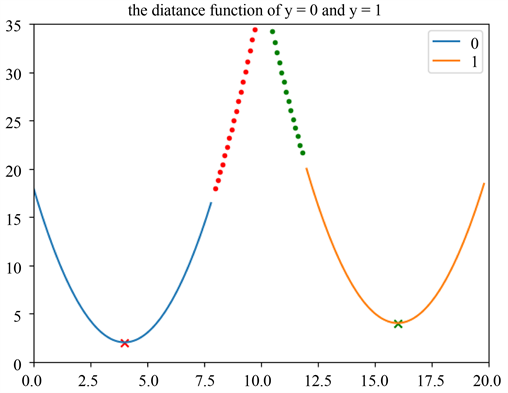Figure 8. Distance function

${\text{distince}}^{k}=\underset{i=1}{\overset{p-q}{\sum }}{\left({x}_{2}^{i}-{x}_{0}^{k}\right)}^{2}-\underset{j=1}{\overset{q}{\sum }}{\left({x}_{2}^{i}-{x}_{0}^{k}\right)}^{2}$ (18)

$\begin{array}{l}\underset{i=1}{\overset{{p}_{2}}{\sum }}{\left({x}_{2}^{1i}-{x}_{0}^{k}\right)}^{2}=\underset{j=1}{\overset{{p}_{1}}{\sum }}{\left({x}_{2}^{0j}-{x}_{0}^{k}\right)}^{2}\\ {x}_{0}^{k}=\frac{\left(2\stackrel{¯}{{x}^{0}}{p}_{1}-2\stackrel{¯}{{x}^{1}}{p}_{2}\right)±\sqrt{{\left(2\stackrel{¯}{{x}^{0}}{p}_{1}-2\stackrel{¯}{{x}^{1}}{p}_{2}\right)}^{2}-4\left({p}_{1}-{p}_{2}\right)N}}{2\left({p}_{1}-{p}_{2}\right)}\\ \stackrel{¯}{{x}^{0}}=\frac{\underset{j=1}{\overset{{p}_{1}}{\sum }}{x}_{2}^{0j}}{{p}_{1}}\\ \stackrel{¯}{{x}^{1}}=\frac{\underset{i=1}{\overset{{p}_{2}}{\sum }}{x}_{2}^{1i}}{{p}_{2}}\\ N=\underset{j=1}{\overset{{p}_{1}}{\sum }}{\left({x}_{2}^{0j}\right)}^{2}-\underset{i=1}{\overset{{p}_{2}}{\sum }}{\left({x}_{2}^{1i}\right)}^{2}\end{array}$ (19)

2.2.4. 基于Sinc函数的逻辑回归算法步骤

1) 对 ${x}_{2×a}$ 进行旋转操作，使得每个 ${x}_{1}$ 上两类 ${x}_{2}$ 数量尽可能相当。

2) 对 ${x}_{1}$ 操作，适当量化，求出尽可能小的自变量间隔，放入T，同时将不同自变量按间隔以从小到大顺序放入 $x{1}_{1×N}$ ，这里假定在间隔T时，有N个不同自变量 $x1$

3) 根据 $x{1}_{1×N}$ ，将 $x2$ 变为 $x{2}_{m×N}$ ，其中m代表每个不同自变量上m个 ${x}_{2}$ 的值。

4) 求出每个 ${x}_{1}$ 上的边界函数上的点，对 ${x}_{2}$ 做如下操作：

for i = 1:N

$x{2}_{m×N}$ 第i列加和除以m，放入x[i]中

end for。

5) 将T带入公式(9)求出相应Sinc函数。

6) 最优点分别与4)中所得Sinc函数相乘并累加，得边界函数。

7) 算法结束。

3. 仿真实验

3.1. 线性回归仿真与对比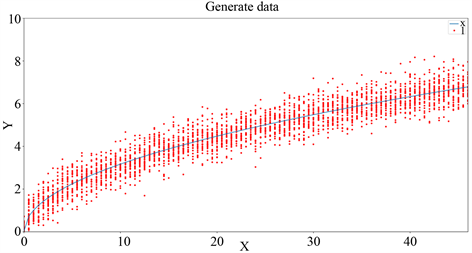Figure 9. Generate data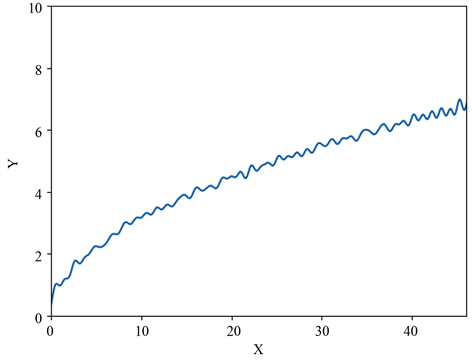Figure 10. Sinc linear regression

3.2. 逻辑回归仿真Table 1. The comparison of two algorithms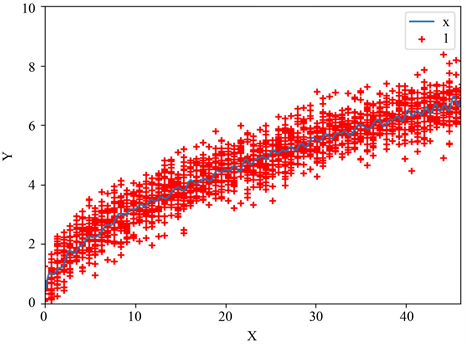Figure 11. Sinc linear regression test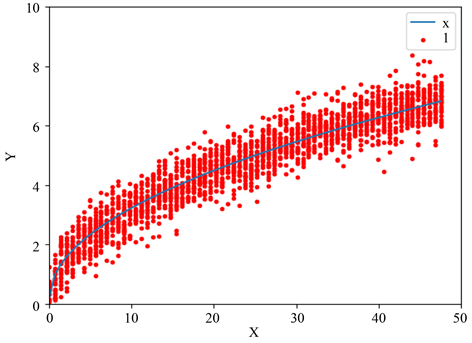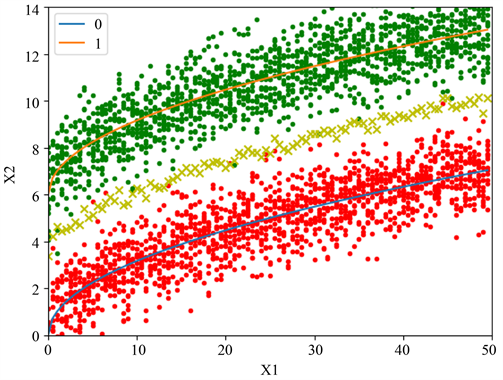Figure 13. Generate data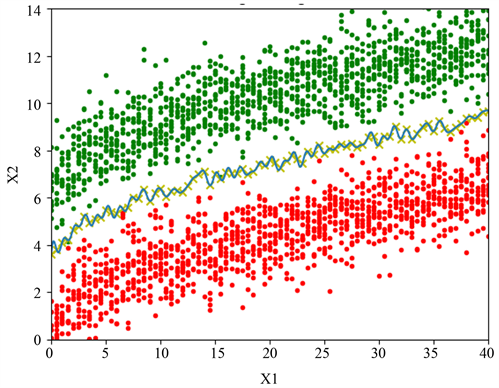Figure 14. Sinc logistic regression

4. 基于Sinc的回归算法的气候预测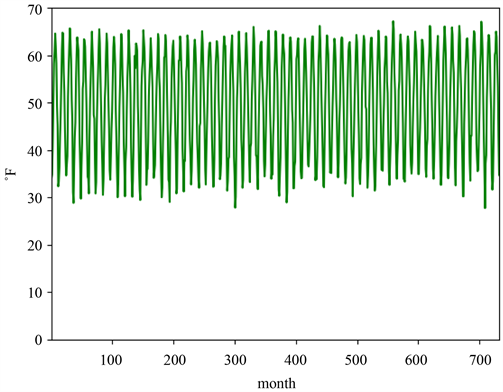Figure 15. Months minimum temperature of 732 data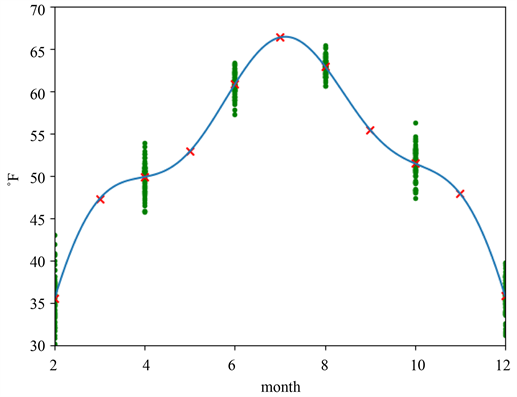Figure 16. TMIN of BIJIE based on Sinc linear regression

5. 结论

1) 在采样定理中离散信号的重构思想启示下，最小化回归函数的均方误差，在数据的自变量域中设计出Sinc函数，然后直接重构出回归曲线；

2) 通过理论推导、算法分析与仿真实验，提出基于Sinc函数的线性与逻辑回归模型及其算法；

3) 将基于Sinc的线性回归算法成功用于月最低气温预测。

 Yahia, M., Hamrouni, T.-A. and Abdelfattah, R. (2017) Infinite Number of Looks Prediction in SAR Filtering by Linear Regression. IEEE Geoscience & Remote Sensing Letters, 14, 2205-2209.
https://doi.org/10.1109/LGRS.2017.2749322

 Watts, B. 非线性回归分析及其应用[M]. 北京: 中国统计出版社, 1998.

 徐全智, 吕恕. 概率论与数理统计[M]. 第二版. 北京: 高等教育出版社, 2010: 201-202.

 Dooley, S.R. and Nandi, A.K. (2000) Notes on the Interpolation of Discrete Periodic Signals Using Sinc Function Related Approaches. IEEE Transactions on Signal Processing, 48, 1201-1203.
https://doi.org/10.1109/78.827555

 Schanze, T. (1995) Sinc Interpolation of Discrete Periodic Signals. IEEE Transactions on Signal Processing, 43, 1502-1503.
https://doi.org/10.1109/78.388863

 Hastie, T., Tibshirani, R. and Friedman, J. (2009) The Elements of Statistical Learning. 2nd Edition, Springer, New York.

 Cervellera, C. and Macciò, D. (2014) Local Linear Regression for Function Learning: An Analysis Based on Sample Discrepancy. IEEE Transactions on Neural Networks & Learning Systems, 25, 2086-2098.
https://doi.org/10.1109/TNNLS.2014.2305193

 Ng, A. (2017) Machine Learning.
https://www.coursera.org/learn/machine-learning

Top